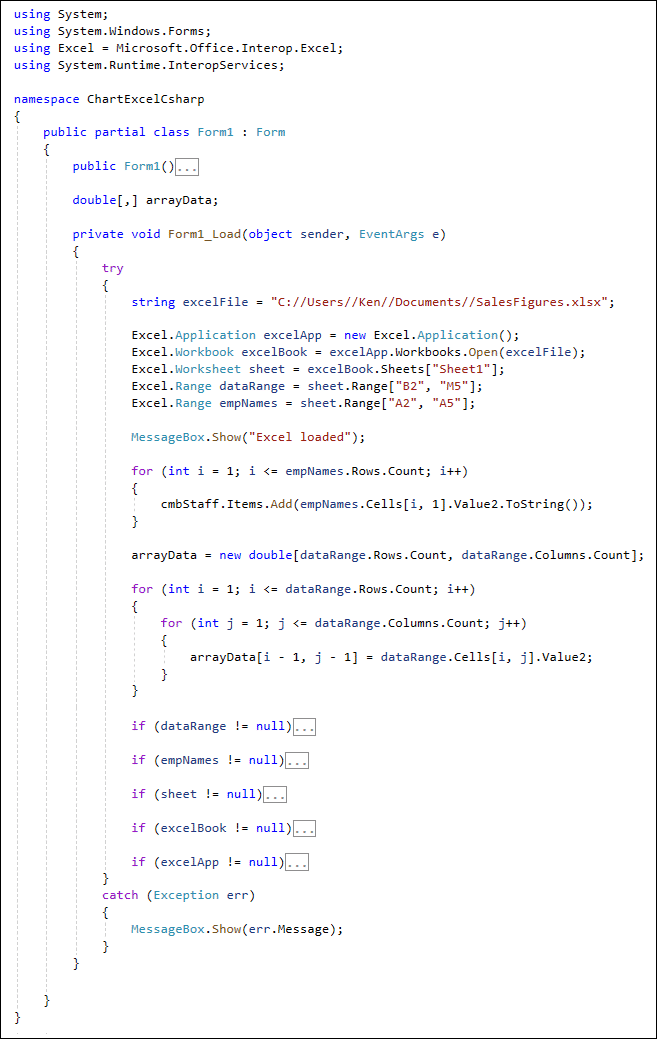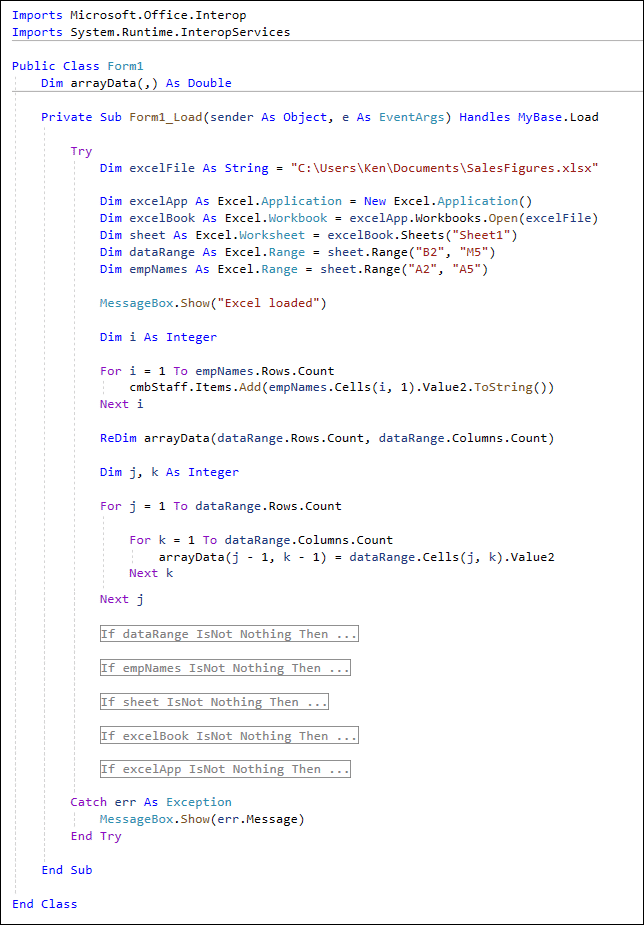# Working with Excel Ranges in C# and VB Net

Excel Chart Project: For C# and VB NET Students

In the previous lesson, we connected to Excel and opened a file. We then grabbed some data from the spreadsheet and placed into two Range objects. In this lessson, we'll do something with that data.

We have the data from the spreadsheet in a Range object called dataRange. The names for the dropdown combo box are in the range object called empNames. The names we can put straight into the combo box using a for loop. However, the data from the dataRange object needs to go into an array. Once it's in an array, it's relatively easy to do data binding for the chart control.

Let's do the combo box first. Add this for loop in C# (add it after MessageBox.Show("Excel loaded") line, but before all those if statements):

for (int i = 1; i <= empNames.Rows.Count; i++)
{

}

Dim i As Integer

For i = 1 To empNames.Rows.Count

Next i

The loop starts at 1. It starts at 1 because Excel values like columns and rows start at 1. The end point for the loop is this:

empNames.Rows.Count

This just gets a count of all the rows in the range called empNames.

Inside the loop, we're adding items to the combo box. Inside of the round brackets of Add, we have this (round brackets after Cells in VB):

empNames.Cells[i, 1].Value2.ToString())

Cells comes from Excel. In between the brackets, you first type the row number you want. After a comma, you type the column number. We're using the loop variable i to loop round all the rows in range. We only have 1 column, so this can be hard-coded. The Value2 part gets the value from the cell in the spreadsheet. You can use just Value, but Value2 is better as it gets the right type of data for you. We then convert the value To a String.

Try it out. Run your program. When the form loads, you should the names in the Combo Box:Now that we have the names from the spreadsheet, the next thing to do is to get all the data and read it onto an array.

## Read an Excel Range in an Array

Set up the following array inside the class Form1: Form line but outside the Form1_Load event in C#:

double[,] arrayData;

In VB, set up the array just after Public Class Form1. Your array is this:

Dim arrayData(,) As Double

We'll need to access this array from elsewhere in the code, which is why it's at the top. But it's a multi-dimensional array to mimic the data from the spreadsheet. This data is in rows and columns, remember. So we can't use an ordinary array. There's only a comma between the brackets of the array meaning it's not set with any sizes. We can do that from the form load event.

In C#, add this line just after your for loop but before the if statements:

arrayData = new double[dataRange.Rows.Count, dataRange.Columns.Count];

ReDim arrayData(dataRange.Rows.Count, dataRange.Columns.Count)

The size of the array is the number of rows in the dataRange range and, after a comma, the number of columns in the range. So, for example, if we have four rows and 12 columns, the array would really be this:

new double[4, 12];

To assign data to the multi-dimensional array, we need a double for loop. Add this in C#:

for (int i = 1; i <= dataRange.Rows.Count; i++)
{

for (int j = 1; j <= dataRange.Columns.Count; j++)
{

arrayData[i - 1, j - 1] = dataRange.Cells[i, j].Value2;

}

}

And this in VB:

For j = 1 To dataRange.Rows.Count

For k = 1 To dataRange.Columns.Count

arrayData(j - 1, k - 1) = dataRange.Cells(j, k).Value2

Next k

Next j

As you're an intermediate student now, we're sure you can figure out how we're filling the arrayData array!

When you've added the loops, your code should look like this in C# (the if statements have been contracted):And this in VB Net:But that's it for the Form Load event. We can do the combo box code, now. We'll get which staff memeber was selected from the list. We'll do that in the next lesson.

ComboBox Code >>

Back to the C# NET Contents Page

Back to the VB NET Contents Page